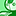# QlikView App Dev

Discussion Board for collaboration related to QlikView App Development.

Announcements
LINKEDIN LIVE: Democratizing data to enhance customer-centricity. JULY 29TH REGISTER TODAY
cancel
Showing results for
Did you mean:Partner

## help with min/max and avg on chart

hi all, i have a chart in the attached qvw which has an expression giving me actuals.

I need to add expressions to show the min/ max and avg but i am not getting much luck in getting it working.

1 Solution

Accepted SolutionsMVP

=Min(Aggr(COUNT(Type),Hour))

=Max(Aggr(COUNT(Type),Hour))

=Avg(Aggr(COUNT(Type),Hour))

=Min(Aggr(Count (Type)/Count(DISTINCT Date),Hour))

=Max(Aggr(Count (Type)/Count(DISTINCT Date),Hour))

=Avg(Aggr(Count (Type)/Count(DISTINCT Date),Hour))

If you want to show for TOTAL.. use as below

=Min(TOTAL......)

=Max(TOTAL.....)

=Avg(TOTAL......)

3 RepliesMVP

=Min(Aggr(COUNT(Type),Hour))

=Max(Aggr(COUNT(Type),Hour))

=Avg(Aggr(COUNT(Type),Hour))

=Min(Aggr(Count (Type)/Count(DISTINCT Date),Hour))

=Max(Aggr(Count (Type)/Count(DISTINCT Date),Hour))

=Avg(Aggr(Count (Type)/Count(DISTINCT Date),Hour))

If you want to show for TOTAL.. use as below

=Min(TOTAL......)

=Max(TOTAL.....)

=Avg(TOTAL......)Master II

Hi,

=Min(Aggr(COUNT(Type),Hour))

=Max(Aggr(COUNT(Type),Hour))

=Avg(Aggr(COUNT(Type),Hour))

And in dimension put:

User

That will give you 3 lin charts for all users.

Regards

KC

Best Regards,
KCMaster II

Sorry, use this:

=Min(Aggr(COUNT(Type),User))

=Max(Aggr(COUNT(Type),User))

=Avg(Aggr(COUNT(Type),User))

And in dimension use:

User

Best Regards,
KCCommunity Browser